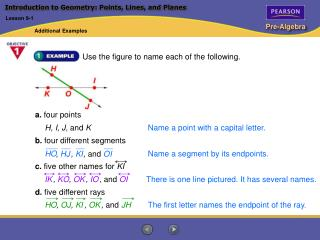Download PresentationIntroduction to Geometry: Points, Lines, and Planes

# Introduction to Geometry: Points, Lines, and Planes

Download Presentation## Introduction to Geometry: Points, Lines, and Planes

- - - - - - - - - - - - - - - - - - - - - - - - - - - E N D - - - - - - - - - - - - - - - - - - - - - - - - - - -
##### Presentation Transcript

1. Use the figure to name each of the following. Name a point with a capital letter. H, I, J, and K HO Name a segment by its endpoints. , HJ , KI , and OI c. five other names for KI , OK , and OI There is one line pictured. It has several names. IK , KO , IO d. five different rays HO The first letter names the endpoint of the ray. , OJ , KI , OK , and JH Introduction to Geometry: Points, Lines, and Planes Lesson 9-1 Additional Examples a. four points b. four different segments

2. MP, OP, QT, ST MQ, NR, OS MN, NO, QR, RS Introduction to Geometry: Points, Lines, and Planes Lesson 9-1 Additional Examples You are looking directly down into a wooden crate. Name each of the following. a. four segments that intersectPT b. three segments parallel to PT c. four segments skew to PT

3. First draw two lines that intersect. Then draw a segment that is parallel to one of the lines. Introduction to Geometry: Points, Lines, and Planes Lesson 9-1 Additional Examples Draw two intersecting lines. Then draw a segment that is parallel to one of the intersecting lines. Use the lines on notebook or graph paper.

4. m 3 + m 4 = 180° 3 and 4 are supplementary. m 3 + 110° = 180° Replace m 4 with 110°. m 3 + 110° – 110° = 180° – 110° Solve for m 3. m 3 = 70° Angle Relationships and Parallel Lines Lesson 9-2 Additional Examples Find the measure of 3 if m 4 = 110°.

5. 1 3, 2 4, 5 7, 6 8 2 7, 6 3 Angle Relationships and Parallel Lines Lesson 9-2 Additional Examples In the diagram, p || q. Identify each of the following. a. congruent corresponding angles b. congruent alternate interior angles

6. Classifying Polygons Lesson 9-3 Additional Examples Classify the triangle by its sides and angles. The triangle has no congruent sides and one obtuse angle. The triangle is a scalene obtuse triangle.

7. Classifying Polygons Lesson 9-3 Additional Examples Name the types of quadrilaterals that have at least one pair of parallel sides. All parallelograms and trapezoids have at least one pair of parallel sides. Parallelograms include rectangles, rhombuses, and squares.

8. Classifying Polygons Lesson 9-3 Additional Examples A contractor is framing the wooden deck shown below in the shape of a regular dodecagon (12 sides). Write a formula to find the perimeter of the deck. Evaluate the formula for a side length of 3 ft. To write a formula, let x = the length of each side. The perimeter of the regular dodecagon is x + x + x + x + x + x + x + x + x + x + x + x. Therefore a formula for the perimeter is P = 12x. P = 12xWrite the formula. = 12(3)   Substitute 3 for x. = 36 Simplify. For a side length of 3 ft, the perimeter is 36 ft.

9. One strategy for solving this problem is to draw a diagram and count the diagonals. A nonagon has nine sides. You can draw six diagonals from one vertex of a nonagon. AH, AG, AF, AE, AD, and AC are some of the diagonals. Problem Solving Strategy: Draw a Diagram Lesson 9-4 Additional Examples How many diagonals does a nonagon have?

10. Problem Solving Strategy: Draw a Diagram Lesson 9-4 Additional Examples (continued) You can organize your results as you count the diagonals. Do not count a diagonal twice. (The diagonal from A to C is the same as the one from C to A.) Then find the sum of the numbers of diagonals. Vertex Number of Diagonals 6 A 6 B 5 C 4 D E 3 F 2 G 1 H 0 I 0 Total 27 A nonagon has 27 diagonals.

11. X , T W , TUV WUX V WX , TU WU , VU XU TV c. Find the length of WX. WX, TV, and TV = 300 m, WX = 300 m. Since Congruence Lesson 9-5 Additional Examples In the figure, TUV WUX. a. Name the corresponding congruent angles. b. Name the corresponding congruent sides.

12. ACB ECD Angle AC EC Side CAB CED Angle by ASA. ACB ECD Congruence Lesson 9-5 Additional Examples List the congruent corresponding parts of each pair of triangles. Write a congruence statement for the triangles. a.

13. MK LJ Side MKJ LJK Angle JK JK Side MKJ LJK by SAS. Congruence Lesson 9-5 Additional Examples (continued) b.